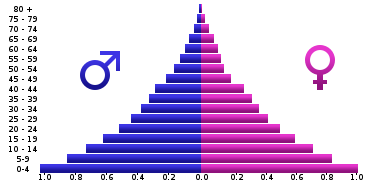Imagine you are in a world where all the parents want to have a boy, and every parent keeps having a children until they have a boy, then they stop giving birth. If the number of male population in this world is given by $p$, and the number of female population is given by $q$. What is the value of $\frac{p}{q}$.